×#### Thank you for registering.

One of our academic counsellors will contact you within 1 working day.

Click to Chat

1800-1023-196

+91-120-4616500

CART 0

• 0

MY CART (5)

Use Coupon: CART20 and get 20% off on all online Study Material

ITEM
DETAILS
MRP
DISCOUNT
FINAL PRICE
Total Price: Rs.

There are no items in this cart.
Continue Shopping• Complete JEE Main/Advanced Course and Test Series
• OFFERED PRICE: Rs. 15,900
• View Details

```Chapter 12: Heron’s Formula Exercise – 12.1

Question: 1

Find the area of a triangle whose sides are respectively 150 cm, 120 cm and 200 cm.

Solution:

Let the sides of the given triangle be a, b, c respectively.

So given,

a = 150 cm

b = 120 cm

c = 200 cm

By using Heron's FormulaSemi perimeter of a triangle = s

2s = a + b + c

s = (a + b + c)/2

s = (150 + 200 + 120)/2

s = 235 cm

Therefore,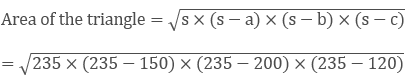= 8966.56 cm2

Question: 2

Find the area of a triangle whose sides are respectively 9 cm, 12 cm and 15 cm.

Solution:

Let the sides of the given triangle be a, b, c respectively.

So given,

a = 9 cm

b = 12 cm

c = 15 cm

By using Heron's FormulaSemi perimeter of a triangle = s

2s = a + b + c

s = (a + b + c)/2

s = (9 + 12 + 15)/2

s = 18 cm

Therefore,= 54 cm2

Question: 3

Find the area of a triangle two sides of which are 18 cm and 10 cm and the perimeter is 42 cm.

Solution:

Whenever we are given the measurements of all sides of a triangle, we basically look for Heron's formula to find out the area of the triangle.

If we denote area of the triangle by A, then the area of a triangle having sides a, b, c and s as semi-perimeter is given by: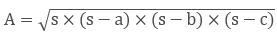Where, s = (a + b + c)/2

We are given:

a = 18 cm

b = 10 cm, and perimeter = 42 cm

We know that perimeter = 2s,

So, 2s = 42

Therefore, s = 21 cm

We know that, s = (a + b + c)/2

21 = (18 + 10 + c)/2

42 = 28 + c

c = 14 cm

So the area of the triangle is: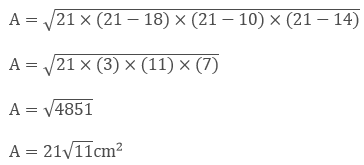Question: 4

In a triangle ABC, AB = 15 cm, BC = 13 cm and AC = 14 cm. Find the area of triangle ABC and hence its altitude on AC.

Solution:

Let the sides of the given triangle be AB = a, BC = b, AC = c respectively.

So given,

a = 15 cm

b = 13 cm

c = 14 cm

By using Heron's FormulaSemi perimeter of a triangle = 2s

2s = a + b + c

s = (a + b + c)/2

s = (15 + 13 + 14)/2

s = 21 cm

Therefore,= 84 cm2

BE is a perpendicular on AC

Now, area of triangle = 84 cm2

1/2 × BE × AC = 84 cm2

BE = 12 cm

The length of BE is 12 cm

Question: 5

The perimeter of a triangular field is 540 m and its sides are in the ratio 25: 17: 12. Find the area of triangle.

Solution:

Let the sides of the given triangle be a = 25x, b = 17x, c = 12x respectively,

So,

a = 25x cm

b = 17x cm

c = 12x cm

Given Perimeter = 540 cm

2s = a + b + c

a + b + c = 540 cm

25x + 17x + 12x = 540 cm

54x = 540 cm

x = 10 cm

Therefore, the sides of a triangle are

a = 250 cm

b = 170 cm

c = 120 cm

Now, Semi perimeter s = (a + b + c)/2

= 540/2

= 270 cm

By using Heron's Formula= 9000 cm2

Therefore, the area of the triangle is 9000 cm2

Question: 6

The perimeter of a triangle is 300 m. If its sides are in the ratio of 3: 5: 7. Find the area of the triangle.

Solution:

Given the perimeter of a triangle is 300 m and the sides are in a ratio of 3: 5: 7

Let the sides a, b, c of a triangle be 3x, 5x, 7x respectively

So, the perimeter = 2s = a + b + c

200 = a + b + c

300 = 3x + 5x + 7x

300 = 15x

Therefore, x = 20 m

So, the respective sides are

a = 60 m

b = 100 m

c = 140 m

Now, semi perimeter= 150 m

By using Heron's Formula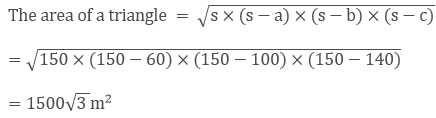Thus, the area of a triangle is 1500√3 m2

Question: 7

The perimeter of a triangular field is 240 dm. If two of its sides are 78 dm and 50 dm, find the length of the perpendicular on the side of length 50 dm from the opposite vertex.

Solution:

Given,

In a triangle ABC, a = 78 dm = AB, b = 50 dm = BC

Now, Perimeter = 240 dm

Then, AB + BC + AC = 240 dm

78 + 50 + AC = 240

AC = 240 - (78 + 50)

AC = 112 dm = c

Now, 2s = a + b + c

2s = 78 + 50 + 112

s = 120 dm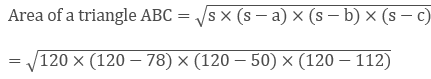= 1680 dm2

Let AD be a perpendicular on BC

Area of the triangle ABC = 1/2 × AD × BC

1/2 × AD × BC = 1680 dm2

Question: 8

A triangle has sides 35 cm, 54 cm, 61 cm long. Find its area. Also, find the smallest of its altitudes?

Solution:

Given,

The sides of the triangle are

a = 35 cm

b = 54 cm

c = 61 cm

Perimeter 2s = a + b + c

2s = 35 + 54 + 61 cm

Semi perimeter s = 75 cm

By using Heron's Formula,= 939.14 cm2

The altitude will be smallest provided the side corresponding to this altitude is longest.

The longest side = 61 cm

Area of the triangle = 1/2 × h × 61

1/2 × h × 61 = 939.14 cm2

h = 30.79 cm

Hence the length of the smallest altitude is 30.79 cm

Question: 9

The lengths of the sides of a triangle are in a ratio of 3: 4: 5 and its perimeter is 144 cm. Find the area of the triangle and the height corresponding to the longest side?

Solution:

Given the perimeter of a triangle is 160m and the sides are in a ratio of 3: 4: 5

Let the sides a, b, c of a triangle be 3x, 4x, 5x respectively

So, the perimeter = 2s = a + b + c

144 = a + b + c

144 = 3x + 4x + 5x

Therefore, x = 12 cm

So, the respective sides are

a = 36 cm

b = 48 cm

c = 60 cm

Now, semi perimeter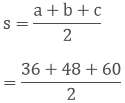= 72 cm

By using Heron's Formula= 864 cm2

Thus, the area of a triangle is 864 cm2

The altitude will be smallest provided the side corresponding to this altitude is longest.

The longest side = 60 cm

Area of the triangle = 12 × h × 60

12 × h × 60 = 864 cm2

h = 28.8 cm

Hence the length of the smallest altitude is 28.8 cm

Question: 10

The perimeter of an isosceles triangle is 42 cm and its base is 3/2 times each of the equal side. Find the length of each of the triangle, area of the triangle and the height of the triangle.

Solution:

Let 'x' be the length of two equal sides,

Therefore the base =1/2 × x

Let the sides a, b, c of a triangle be 1/2 × x, x and x respectively

So, the perimeter = 2s = a + b + c

42 = a + b + c

42 = 3/2 × x + x + x

Therefore, x = 12 cm

So, the respective sides are

a = 12 cm

b = 12 cm

c = 18 cm

Now, semi perimeter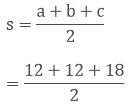= 21 cm

By using Heron's Formula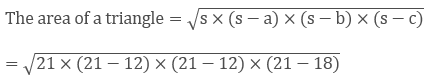= 71.42 cm2

Thus, the area of a triangle is 71.42 cm2

The altitude will be smallest provided the side corresponding to this altitude is longest.

The longest side = 18 cm

Area of the triangle = 12 × h × 18

12 × h × 18 = 71.42 cm2

h = 7.93 cm

Hence the length of the smallest altitude is 7.93 cm

Question: 11

Find the area of the shaded region in fig. belowSolution:

Area of the shaded region = Area of ΔABC − Area of ΔADB

AB2 = AD2 + BD2 .... (i)

Given, AD = 12 cm, BD =16 cm

Substituting the value of AD and BD in eq (i), we get

AB2 = 122 + 162

= 400cm2

AB = 20 cm

Now, area of a triangle = 1/2 × AD × BD

= 96 cm2

Now in triangle ABC,

S =1/2 × (AB + BC + CA)

= 1/2 × (52 + 48 + 20)

= 60 cm

By using Heron's Formula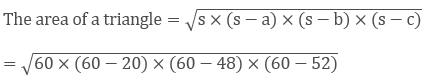= 480 cm2

Thus, the area of a triangle is 480cm2

Area of shaded region = Area of triangle ABC - Area of triangle ADB

= (480 - 96) cm2

= 384 cm2

Area of shaded region = 384 cm2
```### Course Features

• 728 Video Lectures
• Revision Notes
• Previous Year Papers
• Mind Map
• Study Planner
• NCERT Solutions
• Discussion Forum
• Test paper with Video Solution